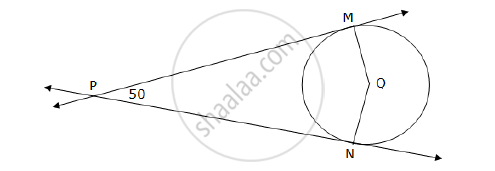SSC (Marathi Semi-English) 10thMaharashtra State Board
Share

# In the following figure, Q is the centre of a circle and PM, PN are tangent segments to the circle. If ∠MPN = 50°, find ∠MQN. - SSC (Marathi Semi-English) 10th - Geometry

ConceptNumber of Tangents from a Point on a Circle

#### Question

In the following figure, Q is the centre of a circle and PM, PN are tangent segments to the circle. If ∠MPN = 50°, find ∠MQN.#### SolutionSeg PM and seg PN are tangents to the circle and seg QM and seg QN are the radii from the points of contacts.

m∠PMQ = m∠PNQ = 90°                  ... (Tangent is perpendicular to the radius) ... (1)

The sum of the measures of the angles of a quadrilateral is 360°.

m∠MPN + m∠PMQ + m∠MQN + m∠PNQ = 360°

50° + 90° + m∠MQN + 90° = 360°

230° + m∠MQN = 360°

m∠MQN = 360° – 230° = 130°                     ... [From (1)]

Is there an error in this question or solution?

#### APPEARS IN

2014-2015 (March) (with solutions)
Question 3.1 | 3.00 marks
Solution In the following figure, Q is the centre of a circle and PM, PN are tangent segments to the circle. If ∠MPN = 50°, find ∠MQN. Concept: Number of Tangents from a Point on a Circle.
S